-->

# STD 4 MATHS ભોપાલનો પ્રવાસ ONLINE UNIT TEST Ncert Solution For Class 4 Maths Chapter 3 Journy of Bhopal

## Overview

### Access Ncert Solution For Class 4 Maths Chapter 3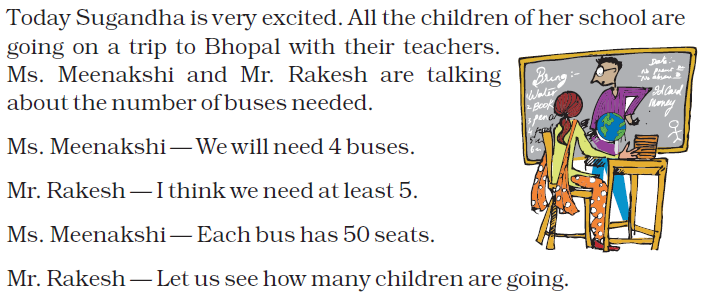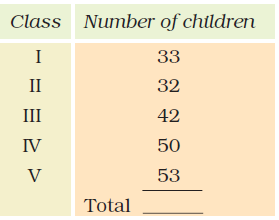Question: 1

Do we need 4 buses? One bus can take 50 children, 4 buses can take 50 × 4 …..?

Answer: If one bus can take 50 children, 4 buses can take 50 × 4 = 200 children.

Question: 2

So, there are a total of ________ children going.

Answer: Number of children going to trip = 33 + 32 + 42 + 50 + 53

= 210

Hence, there are a total of 210 children going

Question: 3

If they get 4 buses, how many children will get seats?

Answer: Total number of seats in 4 buses = Number of seat in a bus × 4

= 50 × 4

= 200

Hence, 200 children will get seats if they get 4 buses.

Question: 4

Will there be any children left without seats?

Answer: Number of students = 210

Number of students who got seats = 200

So, 210 – 200 = 10 children

Therefore, 10 children are left without seats.

NCERT book Page No: 24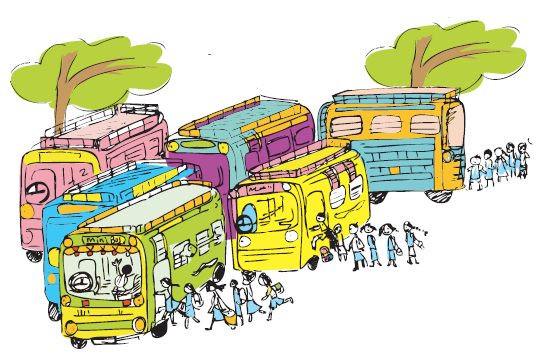Question: 5

Each mini bus can take 35 students. How many mini buses are needed?

Answer: Total number of students going to the trip = 210

Number of students that one mini bus can take = 35

Required number mini buses to take 210 students = 210 ÷ 35

= 6

Therefore, 6 mini buses are required.

NCERT book Page No: 25

Question: 6

If we don’t stop anywhere, we should reach there in 2 hours, that is around ________ O’ clock.

Around 11 O’ clock

Question: 7

If they go to Bhimbetka, they will reach there

– Before 10 O’ clock

– Between 10 O’ clock and 11 O’ clock

– After 11 O’ clock

Answer: If they go to Bhimbetka, they will reach there between 10 O’ clock and 11 O’ clock

NCERT book Page No: 26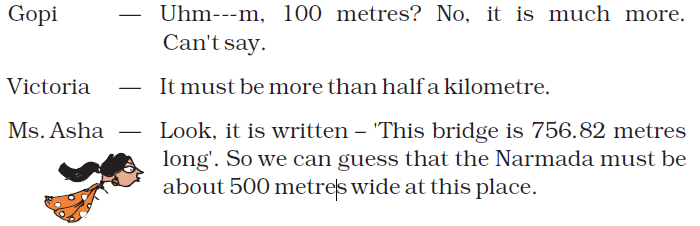Question: 8

Was Victoria right?

Answer: We know that,

1 km = 1000 m

0.5 m = 500 m

The bridge is 756.82 metres long.

So, 756.82 is more than half a kilometre.

Hence, Victoria was right

Question: 8

Have you ever crossed a long bridge? About how many metres long was it?

Answer: Yes, I crossed a long bridge. It was about 756.82 metres long.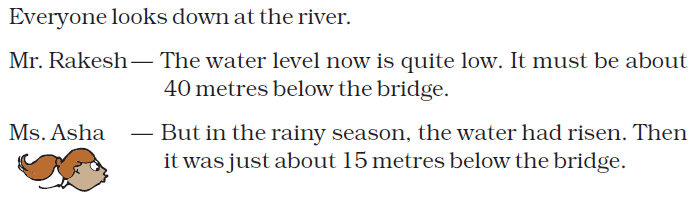Question: 9

What is the difference between the water level of the Narmada in the rainy season and how?

Answer: Difference between the water level of the Narmada in rainy season and now = 40 – 15

= 25 metres

Hence, water level between rainy season and now in Narmada is 25 metres

NCERT book Page No: 27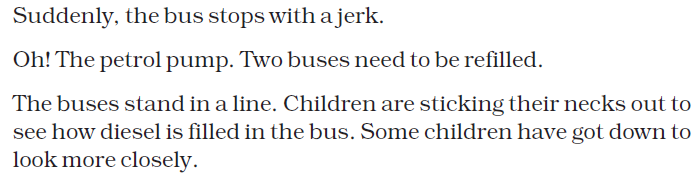Question: 10

Each bus takes about 15 minutes to refill and there are two buses to be refilled. So they stop there for about ____________ minutes, which means they are late by about ________ minutes.

Answer: Time taken to refill 1 bus = 15 minutes

To refill 2 buses = 2 × 15

= 30 minutes

So, they stop there for about 30 minutes, which means they are late by about 30 minutes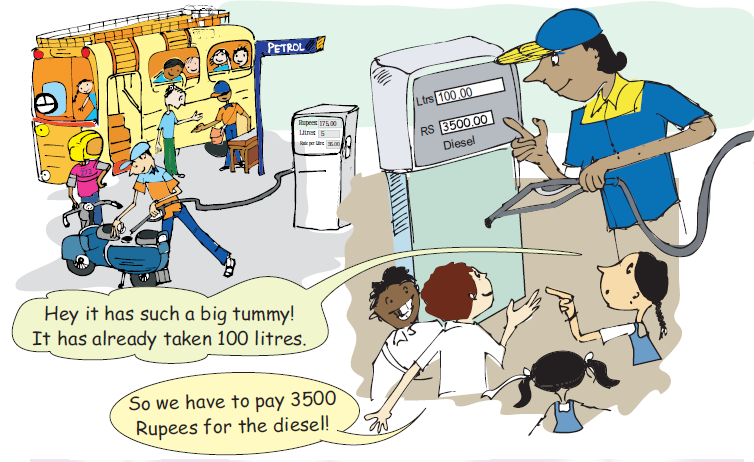Question: 11

Look in the picture and find the price of 1 litres of diesel

Total quantity of diesel filled = 100 litres

The cost of 100 litres of diesel = Rs 3500

Hence, 1 litre of diesel = 3500 ÷ 100 = 35

Therefore, the cost of 1 litre of diesel is Rs 35

As the buses are being refilled, some children go to the toilet near the petrol pump.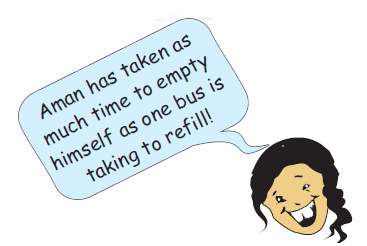Question: 12

How much time did Aman take to come out of the toilet?

Answer: Aman took 15 minutes to come out of the toilet

NCERT book Page No: 28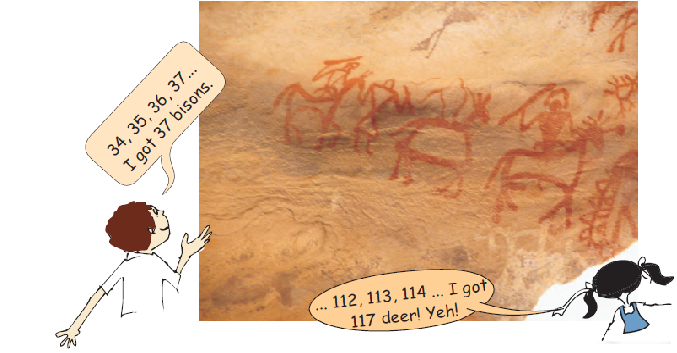Question: 13

How many more deer are there than bisons?

Answer: Number of deer = 117

Number of bisons = 37

Number of deer more than bison = Number of deer – Number of bison

= 117 – 37

= 80

So, there are 80 more deer than bison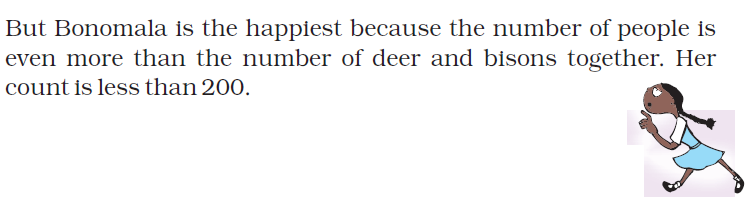Question: 14

How many people must Bonomala have counted?

214 / 154 / 134 / 177

Answer: Total number of animals = 117 deer + 37 bisons

= 154

Number of people given is less than 200. So, number of people must be greater than 154 but less than 200

Hence, Bonomala must have counted 177 people

Question: 15

They have spent 1 hour there. What time is it?

Answer: Since it is given that they reached Bhimbetka at 11 O’clock.

So, 11 O’ clock + 1 hour = 12 O’ clock

Therefore, it is 12 O’ clock.

Question: 16

They are now moving towards Bhopal. They should reach there in less than 1 hour, at about __________ O’ clock.

Answer: At 12 O’ clock they leave from Bhimbetka. They are now moving towards Bhopal. Thus they should reach there in less than 1 hour, i.e., at about 1 O’clock.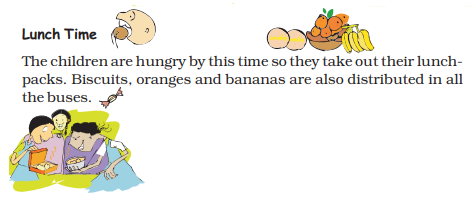Question: 17

Each child is to be given 1 orange, 1 banana and 5 biscuits. All the children take oranges and biscuits but 38 children do not take bananas. How many oranges, biscuits and bananas are distributed?

Answer: Total number of students going to a trip = 210

Each students was given 1 orange, 1 banana and 5 biscuits.

Hence, total number of oranges distributed to 210 students = 1 × 210 = 210

Since, 38 students refused to take bananas. Then total number of students who took bananas = 210 – 38

= 172

Therefore, total number of bananas distributed to 210 students = 210 × 5

= 1050

Thus, 210 oranges, 172 bananas and 1050 biscuits were distributed among all the students going to the trip

Manjeet and Bhanu quickly finish their lunch and start asking puzzles to pass the time.

Question: 18

Tell me the number which is exactly between 100 and 150

Answer: 125 is the number which is exactly between 100 and 150

Question: 19

I gave four toffees each to four of my friends and three toffees are left with me. How many toffees did I have?

Answer: Given number of toffees given to 4 friends = 4

Number of toffees left with me = 3

Total number of toffees = number of toffees I gave to my 4 friends + Number of toffees left with me

= 4 × 4 + 3

= 16 + 3

= 19 toffees

Therefore total number of toffees I have = 19 toffees.

Question: 20

What number can you make using 3, 5 and 7? You can make 357 and 537. What others?

Answer: The other number made using 3, 5 and 7 are 375, 573, 735 and 753

Question: 21

A number becomes double if it is increased by 8. What is the number?

Answer: 8 + 8 = 16 which is double of 8

Therefore the number will be 8

Question: 22

Think of a number which can be divided by 2, 3 and 5 and comes between 25 and 50.

Answer: The numbers between 25 and 50 that are divisible by 2 are 26, 28, 30, 32, 34, 36, 38, 40, 42, 46 and 48

The numbers between 25 and 50 that are divisible by 3 are 27, 30, 33, 36, 39, 42, 45 and 48

The numbers between 25 and 50 that are divisible by 5 are 30, 35, 40 and 45

Hence, the smallest number between 25 and 50 that can be divisible by 2, 3 and 5 is 30

Question: 23

A small ant climbs 3 cm in 1 minute but slips down 2 cm. How much time will it take to climb to 2 cm?

Answer: Given that the small ant climbs 3 cm in 1 minute and slips down 2 cm.

Hence, distance covered by the ant in 1 minute = 3 – 2

= 1 cm

Therefore, the ant will take 2 minutes to cover a distance of 2 cm.

NCERT book Page No: 32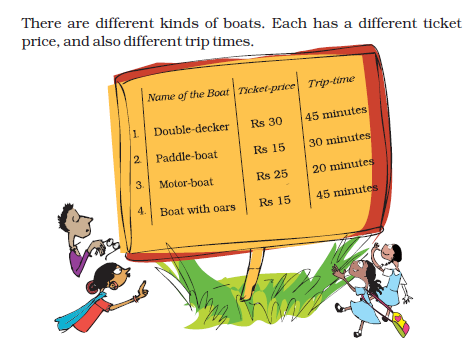NCERT book Page No: 33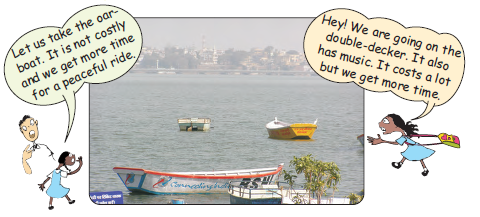Question: 24

Indra and Bhanu first went in the motor – boat, and then took the oar – boat.

How much did they pay for both the boats? Rs ________

How much time did they get for both rides? __________

Answer: Total money Indra and Bhanu paid for the motor boat = Rs 25 + Rs 25 = 50

Money paid by them for the oar boat = Rs 15 + Rs 15 = Rs 30

Total money Indra and Bhanu paid for the motorboat and the oar boat = Rs 50 + Rs 30 = Rs 80

Time for the motorboat ride = 20 minutes

Time for the oar boat ride = 45 minutes

Total time for both the rides = 20 + 45 = 65 minutes

Question: 25

One group of children went for the double – decker trip. They paid Rs 450 in total. How many children went for the double – decker trip? _________

Answer: Money spent by the group of children for the double decker trip = Rs 450

Price paid for the double decker trip per child = Rs 30

Number of children went for the double decker trip = total rupees paid / ticket price per child

= Rs 450 / 30

= 15 children

Question: 26

Which boat makes two trips in 1 hour?

Answer: We know 1 hour = 60 minutes

From the table, paddle boat takes 30 minutes to complete a trip. Hence, paddle boat will take 2 trips in 60 minutes i.e., 1 hour

Question: 27

Which boat takes less than half an hour to complete a trip?

Answer: We know half an hour = 30 minutes

From the table, motor boat takes 30 minutes to complete a trip. Hence, the motor boat takes less than 30 minutes to complete a trip.

Question: 28

Which boat gives them the most time taking the least money?

Answer: From the table, we come to know that the least money, i.e., Rs 15 is charged by the paddle boat and the oar boat. Here, the oar boat trip gives more time compared to that given by the paddle boat for the same amount. Therefore oar boat gives the most time taking the least money.

Question: 29

Javed went twice for boating. He paid a total of Rs 40 and boated for 50 minutes. Which two boats did he take?

Answer: Javed took paddle and motor boat

Total money paid by him = Rs 40

Total time spent by him = Rs 50 minutes

From the table, we can see that Javed took the paddle boat and motor boat trips, which together cost him Rs 40 and the total time spent on both the boats is 50 minutes.

Time to return

Question: 30

Children enjoy different boat rides till 4 O’clock. It is time to return. Now they will not stop anywhere and reach back in two hours. So, they should reach Hoshangabad by ____________ O’ clock

Answer: Starting time + Time take to reach

= 4 O’clock + 2 hours

= 6 O’ clock

They should reach Hoshangabad by 6 O’ clock

Practice Time

Question: 31

There are four very old cave paintings. Mark the oldest.

(a) 4200 years old

(b) 1000 years old

(c) 8500 years old

(d) 1300 years old

Answer: Among all the cave paintings, 8500 years is the oldest cave painting. Hence option (c) is the correct.

Question: 32

One bus can carry 48 children. How many children can three buses carry? About –

(a) 100

(b) 200

(c) 150

Answer: Number of children in a bus = 48 children

Number of children three buses can carry = 48 × 3

= 144

So, there are about 150 children

Therefore, option (c) is the correct

Question: 33

Which pair of numbers add to make more than 500?

(a) 152 and 241

(b) 321 and 192

(c) 99 and 299

(d) 401 and 91

Answer: Let us add the pairs to check the pair that has the sum greater than 500

152 + 241 = 393

321 + 192 = 513

99 + 299 = 398

401 + 91 = 492

Therefore, 321 + 192 gives 513 which is more than 500. So, option (b) is the correct answer.

Question: 34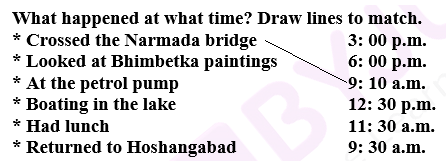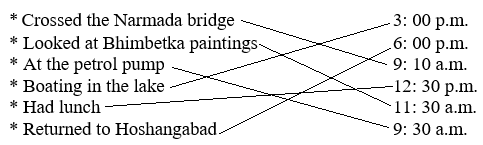NCERT Class 4 maths chapter 3 is one of the most exciting chapters in the syllabus, containing Maths topics related to Bhopal trip. With questions regarding the trip to Bhopal and the bus trip with the children filled in it, this adventurous chapter brings along a series of questions and easy to solve topics that will boost up your concentration when it comes to observing details.

The NCERT Solutions Class 4 Maths Chapter 3 – A Trip To Bhopal contains example such as;

• Find the number of oranges, biscuits, etc. distributed
• A small Ant climbs two steps slides back down two steps, total how many steps covered.

The above were a few questions from Chapter 3 Class 4 syllabus.

Download, BYJU’S-The Learning app to get personalized videos based on the topics explained in each chapter of the 4th standard Maths subject.

## Frequently Asked Questions on NCERT Solutions for Class 4 Maths Chapter 2

### What are the different kinds of boats mentioned in Chapter 2 of NCERT Solutions for Class 4 Maths?

There are 4 different kinds of boats and each has a different ticket price, and also different trip times. The boats are:
1. Double-decker costing Rs 30 per ticket for a duration of 45 min
2. Paddle boat costing Rs 15 per ticket for a duration of 30 min
3. Motor boat costing Rs 20 per ticket for a duration of 20 min
4. Boat with oars costing Rs 15 per ticket for a duration of 45 min

### Think of a number that can be divided by 2, 3 and 5 and comes between 25 and 50 as discussed in Chapter 2 of NCERT Solutions for Class 4 Maths.

A number that can be divided by 2, 3 and 5 should be a multiple of their product. The product of 2, 3 and 5 is 30. Now, the number 30 is also lying between 25 and 50. Hence, the required number is 30.

### Related Posts

There is no other posts in this category.

1.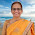1.